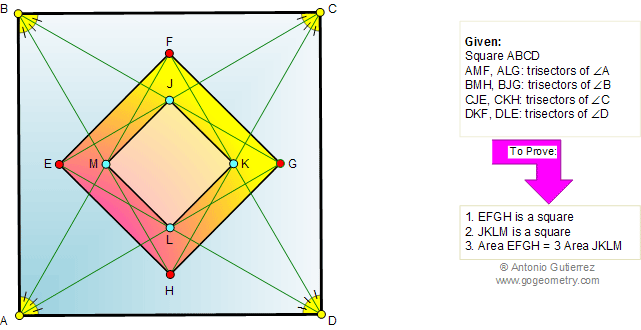Problem 396: Square, Angle Trisectors, Congruence, Area

 The figure shows a square ABCD with lines AMF, ALG, BMH, BJK, CJE, CKH, DKF, and DLE the trisectors of angles A, B, C, and D, respectively. Prove that (1) EFGH is a square, (2) JKLM is a square, and (3) the area of square EFGH is 3 times the area of square JKLM. .Home | Search | Geometry | Problems | All Problems| 391-400 | Square area | Area of a Parallelogram | Email | View or post a solution